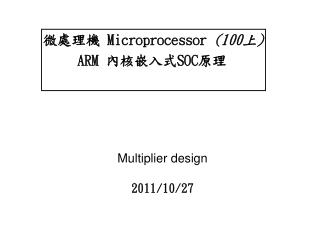DownloadDownload PresentationMultiplier design 2011/10/27

# Multiplier design 2011/10/27

Télécharger la présentation## Multiplier design 2011/10/27

- - - - - - - - - - - - - - - - - - - - - - - - - - - E N D - - - - - - - - - - - - - - - - - - - - - - - - - - -
##### Presentation Transcript

1. Multiplier design2011/10/27 微處理機 Microprocessor (100上) ARM 內核嵌入式SOC原理

2. One bit Multiplier for ARM1 • Since the 32-bit addition time has a significant effect on the datapath cycle time • Maximum clock rate and the processor's performance

3. One bit Multiplier

4. B A = 1 0 0 1 * 1 1 0 1 3 2 1 0 N TURN N=0： MUL=1 A = A + (B LSL 0) A = 0 + 1001 N=1： MUL=0 A = A + 0 A = 1001 + 0 = 1001 N=2： MUL=1 A = A + (B LSL 2) A = 1001 + 100100 = 101101 N=3： MUL=1 A = A + (B LSL 3) A = 101101 + 1001000 = 1110101

5. Multiplier design • All ARM processors apart from the first prototype have included hardware support for integer multiplication. Two styles of multiplier have been used • Older ARM cores include low-cost multiplication hardware that supports only the 32-bit result multiply and multiply-accumulate instructions • Recent ARM cores have high-performance multiplication hardware and support the 64-bit result multiply and multiply-accumulate instructions

6. Booth algorithm • Two bit multiplier • This allows all four values of the 2-bit multiplier to be implemented by a simple shift and add or subtract • x 3 =[ x (-1) + x 4 ] • carrying the x 4 over to the next cycle

7. modified Booth's algorithm

8. B A = 10110001 * 11010011 3 2 1 0 N TURN N=0： Cin=0 MUL=11 A = A - (B LSL 0) set Cout = 1 A = 0 + 1111111101001111 N=1： Cin=1 MUL=00 A = A + (B LSL 2) set Cout= 0 A = 1111111101001111 + 0000001011000100 = 0000001000010011 N=2： Cin=0 MUL=01 A = A + (B LSL 4) set Cout = 0 A = 1000010011 + 101100010000 = 0000110100100011 N=3： Cin=0 MUL=11 A = A - (B LSL6) set Cout = 1 A = 110100100011 + 1101001111000000 = 1110000011100011 N=4： Cin=1 MUL=00 A = A + (B LSL 8) set Cout = 0 A = 1110000011100011 + 1011000100000000 = 1001000111100011

9. 實習問題 實習一: 使用One bit Multiplier將 10 11 00 01 * 11 01 00 11 的二進制乘法用組合語言寫出。 實習二: 使用Booth‘s algorithm將 10 11 00 01 * 11 01 00 11 的二進制乘法用組合語言寫出。 做完請找助教檢查